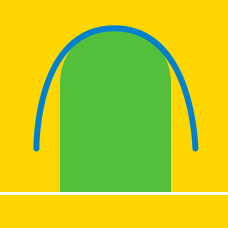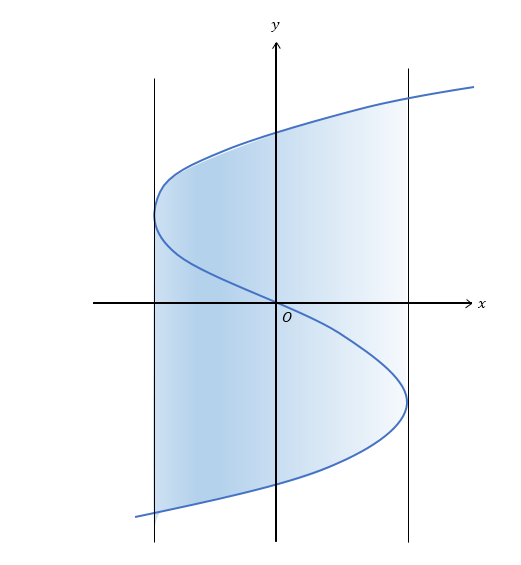Calculus

# Area between Curve and y-axisIf the blue curve in the above diagram represents the graph of $$x={y}^3-300y$$ and the vertical lines are tangent lines to the curve, what is the area of the shaded region?

What is the area of the region bounded by the curve $$x= -{y}^2+100$$ and the $$y$$-axis ?

Let $$S$$ be the area bounded by $$y^2 \leq x$$, $$x \leq 25$$ and $$y \geq 0$$. If $$S = \frac{a}{b}$$, where $$a$$ and $$b$$ are coprime positive integers, what is the value of $$a+b$$?

What is the area of the region bounded by the curve $$x= -6y(y-4)$$ and the $$y$$-axis ?

If $$g(x)$$ is the inverse function of $$f(x)=10\cos x$$ $$(0 \leq x \leq \frac{\pi}{2}) ,$$ what is the area of the region bounded by $$y=g(x) ,$$ the $$x$$-axis and $$y$$-axis?

×